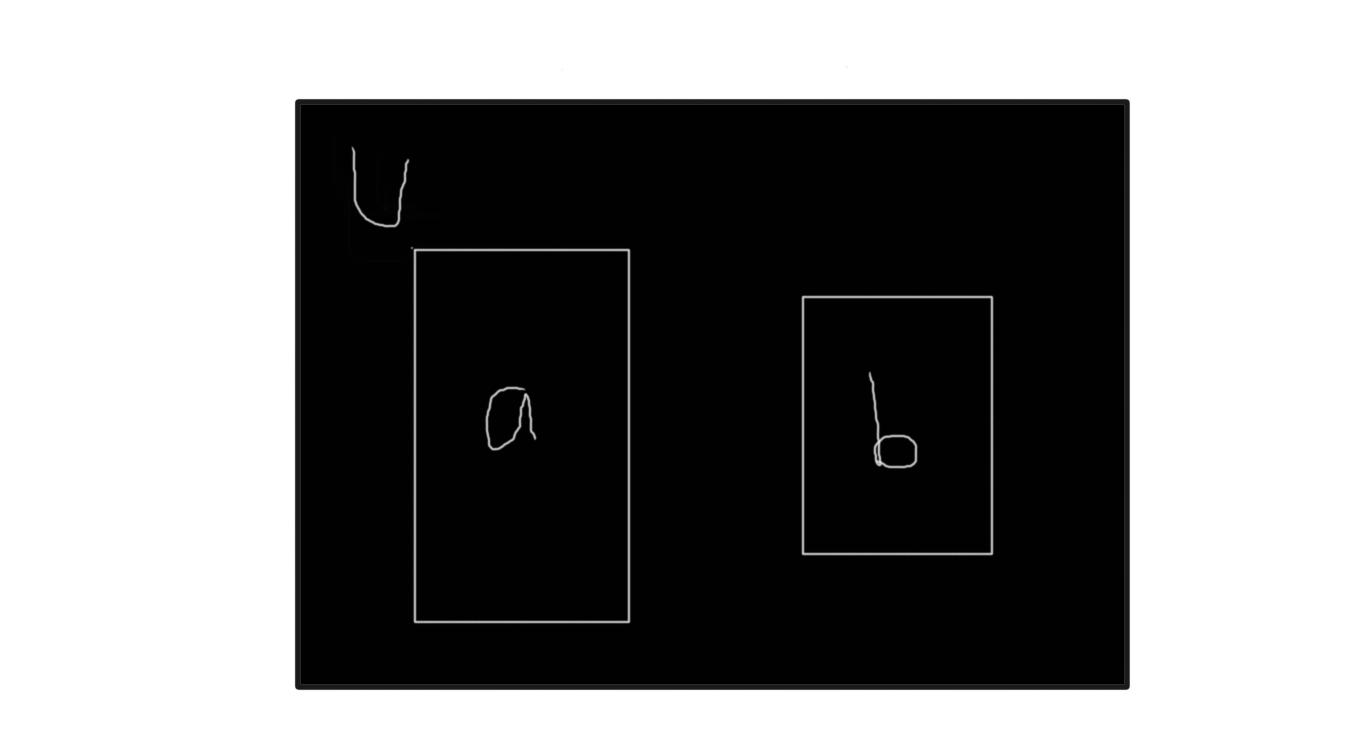# Introduction

With De Morgan's law and truth tables, we will be able to simplify logical expressions and models, find possibilities and even bugs. These processes help us organize, simplify and almost even visualize how things will work. Truth tables are also useful in designing logic circuits and logic gates. Let's dive in.

# De Morgan's Law

The complement of the union of two sets is the intersection of their complements and the complement of the intersection of two sets is the union of their complements.

That's a lot of gibberish, I know, but I understood this personally with this exampleIf U is {1,2,3,4,5,6}, A is {2,3} and B is {3,4,5}

``````//The union of A and B
A u B = {2,3,4,5}

// What the universal set contains and (A u B) doesn't
(A u B)' = {1,6}

// What the universal set contains and A doesn't
A' = {1,4,5,6}

//What the universal set contains and B doesn't
B' = {1,4}

//The intersection of the complements of A and B
A' n B' = {1,6}

A u B = A' n B'``````

In English, It can't be summer and winter at once, so its either not summer or not winter. And it's not summer and not winter if and only if it's not the case that its either summer or winter. Following this reasoning, ANDs can be transformed into ORs and vice versa

This basically means

``````/*it cannot be summer and winter at once also
means it is either, not summer or not winter,
using the template that its either summer or winter that are available*/

!(Summer AND Winter) = !Summer OR !Winter

/*If its either not summer and not winter, that means it can't be summer or winter*/

!Summer AND !Winter = !(Summer OR Winter)``````

# Truth tables

We can use truth tables to analyze the internal variables that our model relies on. The rows represent the possible states or combinations for the variables. Each variable has two possible outcomes, so we use the 2 ^ n formula, where n is the number of variables. Each outcome can either be True or False.

# Use Case

``````class User {
constructor(firstname, lastname, isValidated, rateCount,isBlocked){
this.firstname = firstname;
this.lastname = lastname;
this.isValidated = isValidated;
this.rateCount = rateCount;
this.isBlocked = isBlocked;
}

writeToFile() {
if(!this.isBlocked && this.rateCount < 10 && this.isValidated ){
console.log('User is writing...');
}else console.log(`\${this.firstname} \${this.lastname} you have issues`)

}

this.rateCount ++;
}

get rate(){
return this.rateCount;
}

}``````

This is a system that grants write permissions to authenticated users and blocks users if they try to write to the system with an unvalidated email address or tries to write to the system after the limit of 10 commits is exceeded.

Using De Morgan's Law

We want to analyze the logical path or process that leads to a user getting blocked.

A: Unvalidated User
B: Writes to the system
C: Exceeding rate limit(10)
D: User gets blocked

``````/*If an Unvalidated user writes to the system or if a validated user exceeds the limit, the user gets blocked.*/
(A AND B) OR (B AND C) -> D

//We can factorize using distributivity
B AND (A OR C) -> D

//The user remains unblocked at !D
!D -> !(B AND (A OR C)) // The law of contrapositivity

//Using DeMorgan's law (!(A AND B) = !A OR !B)
!D -> !B OR !(A OR C)

//Using DeMorgan's law again
!D -> !B OR (!A AND !C)``````

The final expression tells us that the user is not blocked if he doesn't write to the system or if he is validated and doesn't exceed the limit.

Using Truth tables

If we have to create a system with the following requirements

• If the user hasn't validated his/her email, he/she has only read permissions.
• An Unvalidated user cannot have write permissions
• The user either has read or write permissions.

A: Unvalidated User
C: write permissions

1. A ---> B (Unvalidated User has only read permissions )
This statement is only true, when the output(B) is true, or when they are both(A and B) false.
2. !(A and C) (An Unvalidated User can't have write permissions)
3. B or C (It's either a user has read or write permissions)

Since we have three variables, we will have 8 possible outcomes (2 ^ 3) of true or false for each variable. Then we test these possible outcomes with the three statements we have above.

A B C 1 2 3 Total
T T T T F T F
T T F T T T T
T F T F F T F
T F F F T F F
F F F T T F F
F F T T T T T
F T T T T T T
F T F T T T T

So we can see that we only have truthy outcomes when at least one of the variables is false, or at least one of the variables is true. They can't be all false or all true. Which makes sense, you can't be invalidated and still have to write permissions.

# Conclusion

There are so many other rules and laws that come with analyzing logical models, this is just a slice of the pie, I came across it and I liked it, so I decided to share. Thanks for reading! 🌹 🌹

1. Computer Science Distilled by Wladston Ferreira Filho